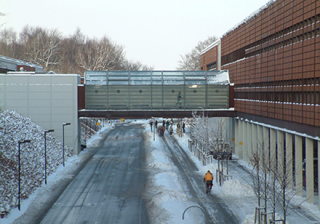# Physics and mathematics: methods and modelsThe overall goal of the course is to bridge the gap between basic mathematical techniques and their applications within science, physics in particular. In the physics part of the course, the students learn to use physical laws to formulate mathematical models, solve these models and evaluate the results with due attention paid to the limitations of the models. The students will furthermore carry out experiments that illustrate the theory, and learn to interpret the results. The mathematical part of the course will train the students to deal with scientific models by identifying and applying the relevant mathematical methods, including mathematical symbolic language and logical arguments. More information about the course is available here.

Date
09-02-2015
15-12-2015
Institution
University of Southern Denmark, Department of Physics Chemistry and Pharmacy
Role
Tutorial Assistant
Teacher
Emil Sjulstok Rasmussen